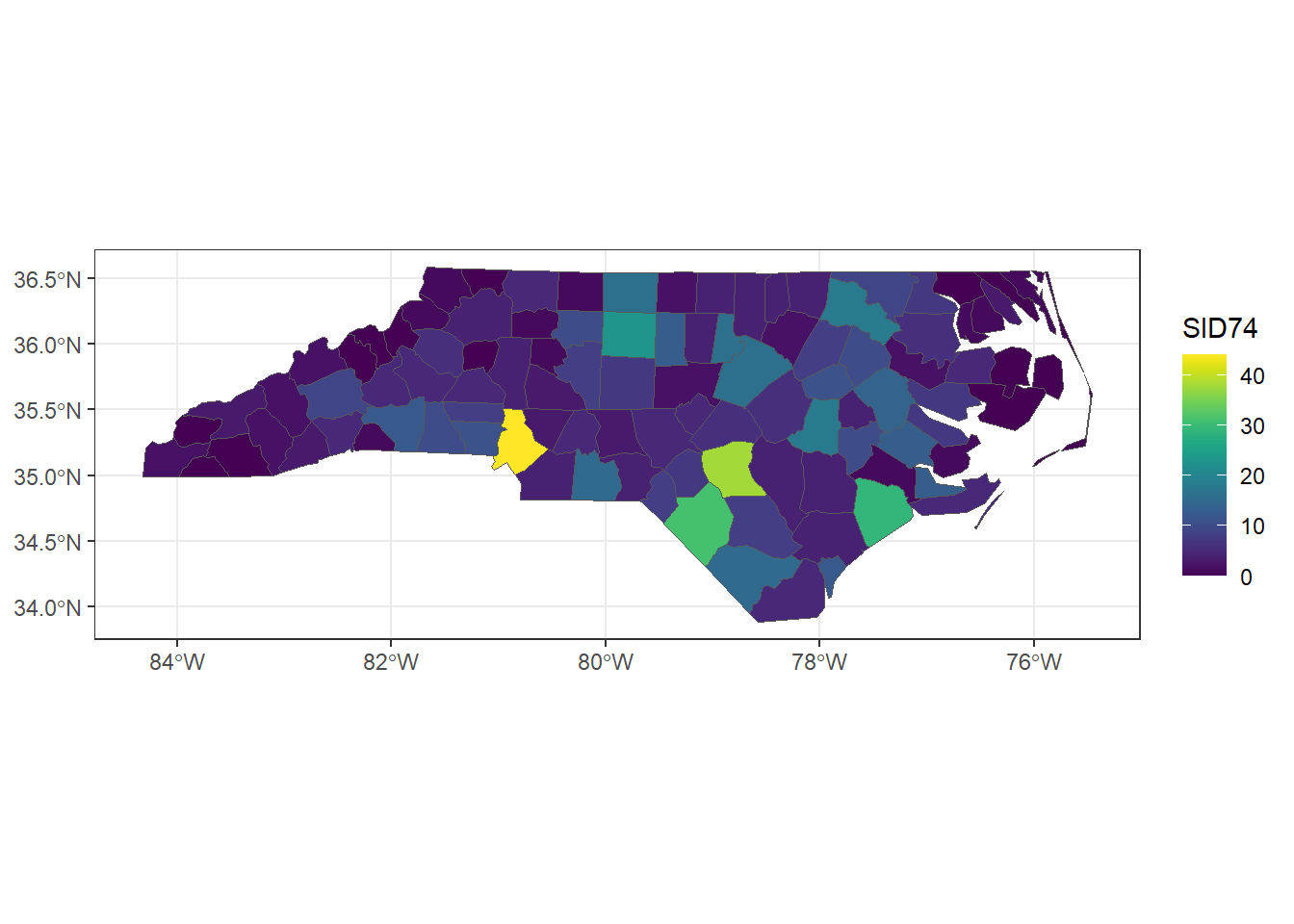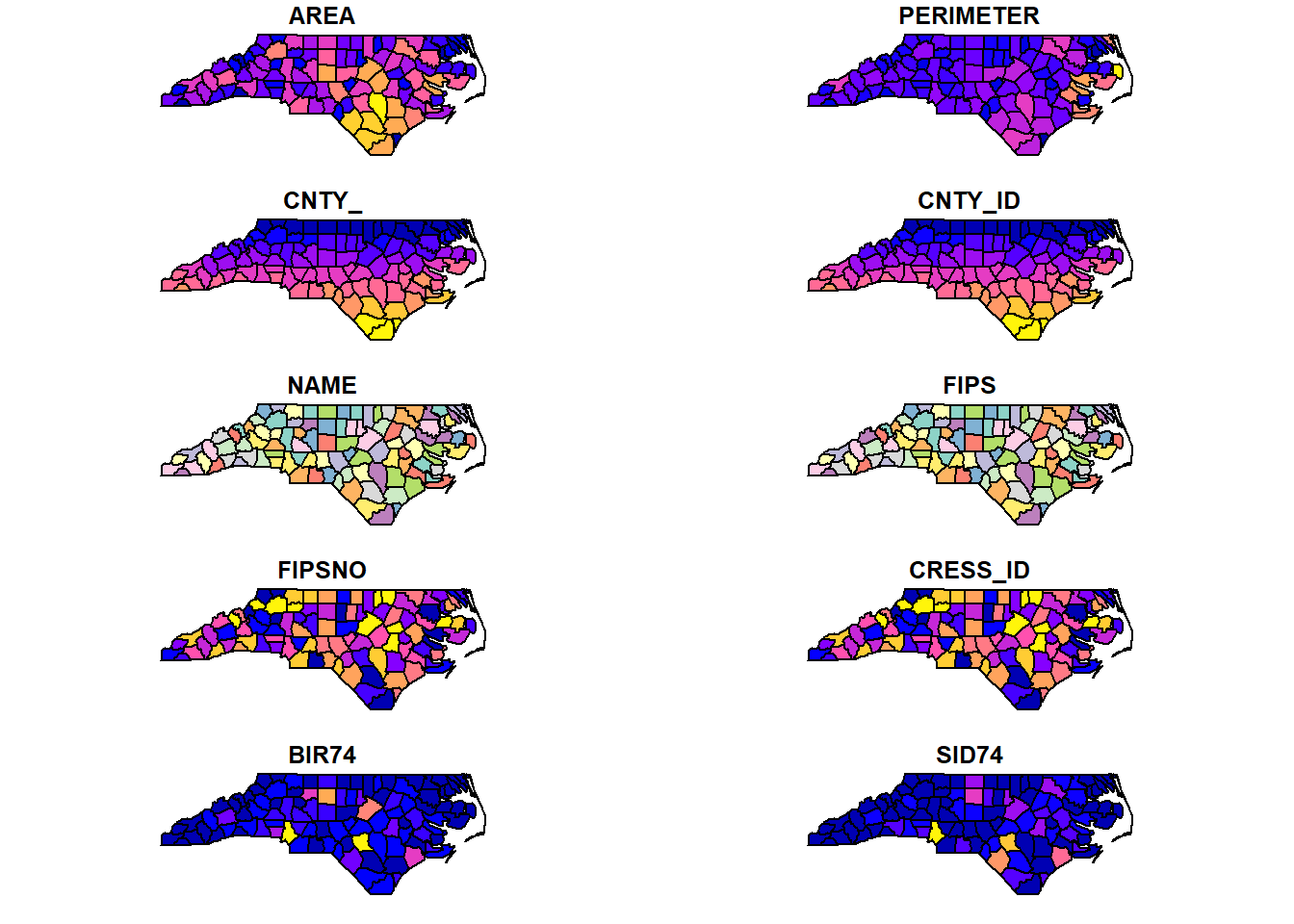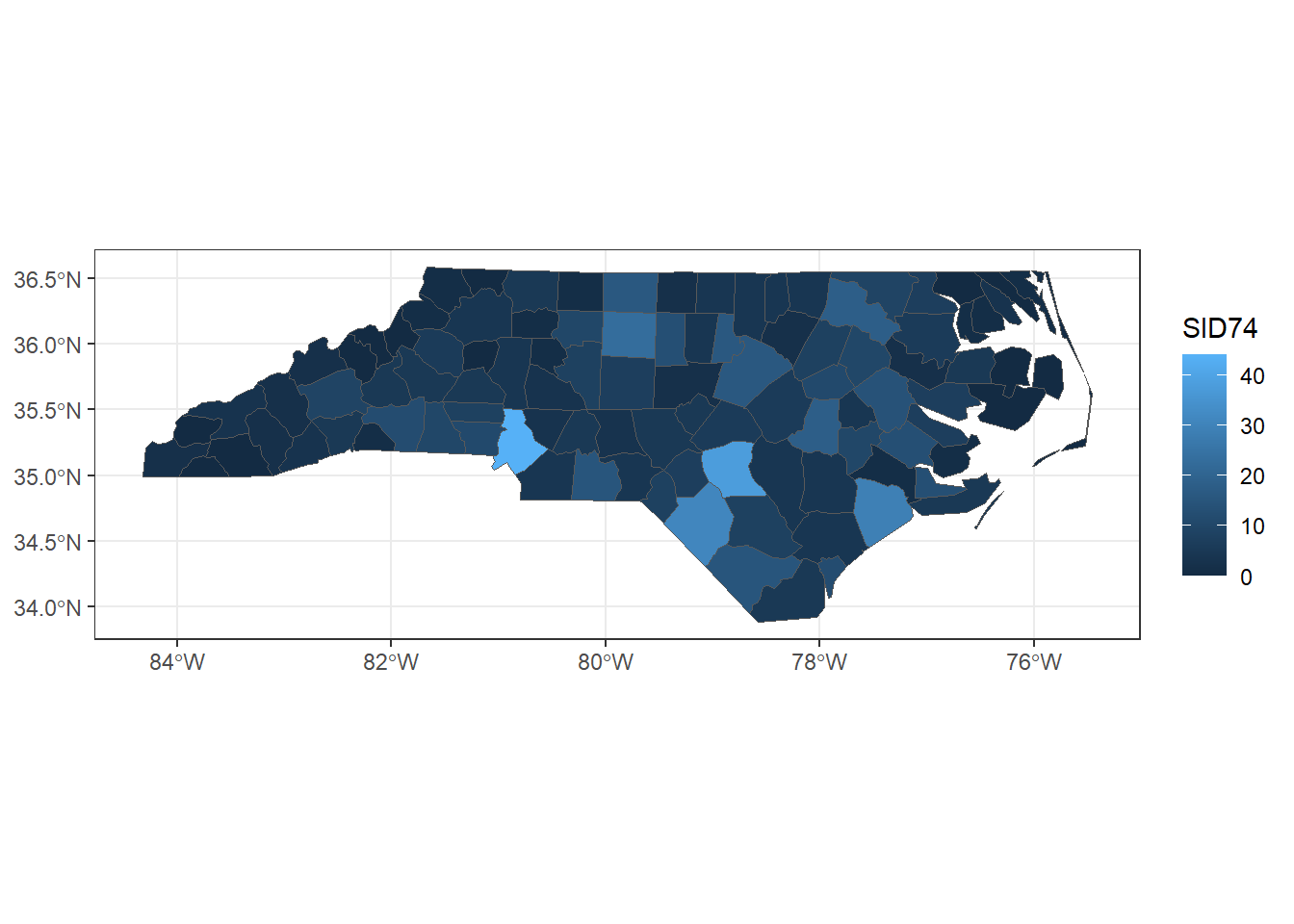# 2 Spatial data and R packages for mapping

In this chapter we describe the basic characteristics and provide examples of spatial data including areal, geostatistical and point patterns. Then we introduce the geopraphic and projected coordinate reference systems (CRS) that are used for spatial data representation, and show how to use R to set CRS and transform data to different projections. Then we describe the data storage format called shapefile to store geospatial data. Finally, we present several examples that show R packages useful to create static and interactive maps including ggplot2 , leaflet , mapview and tmap . Examples show how to define color palettes, create legends, use background maps, plot different geometries, and save maps as HTML files or as static images.

## 2.1 Types of spatial data

A spatial process in $$d=2$$ dimensions is denoted as

$\{ Z(\boldsymbol{s}): \boldsymbol{s} \in D \subset \mathbb{R}^d\}.$

Here, $$Z$$ denotes the attribute we observe, for example, the number of sudden infant deaths or the level of rainfall, and $$\boldsymbol{s}$$ refers to the location of the observation. distinguishes three basic types of spatial data through characteristics of the domain $$D$$, namely, areal data, geostatistical data, and point patterns.

### 2.1.1 Areal data

In areal (or lattice) data the domain $$D$$ is fixed (of regular or irregular shape) and partitioned into a finite number of areal units with well-defined boundaries. Examples of areal data are attributes collected by ZIP code, census tract, or remotely sensed data reported by pixels.

An example of areal data is shown in Figure 2.1 which depicts the number of sudden infant deaths in each of the counties of North Carolina, USA, in 1974 . Here the region of interest (North Carolina) has been partitioned into a finite number of subregions (counties) at which outcomes have been aggregated. Using data about population and other covariates, we could obtain death risk estimates within each county. Other examples of areal data are presented in where Bayesian hierarchical models are used to estimate the relative risk of low birth weight in Georgia, USA, in 2000, and where spatial variations in temporal trends are assessed to detect unusual cervical cancer trends in white women in the USA over the period from 1969 to 1995.

library(sf)
library(ggplot2)
library(viridis)
nc <- st_read(system.file("shape/nc.shp", package = "sf"),
quiet = TRUE
)
ggplot(data = nc, aes(fill = SID74)) + geom_sf() +
scale_fill_viridis() + theme_bw()FIGURE 2.1: Sudden infant deaths in North Carolina in 1974.

### 2.1.2 Geostatistical data

In geostatistical data the domain $$D$$ is a continuous fixed set. By continuous we mean that $$\boldsymbol{s}$$ varies continuously over $$D$$ and therefore $$Z(\boldsymbol{s})$$ can be observed everywhere within $$D$$. By fixed we mean that the points in $$D$$ are non-stochastic. It is important to note that the continuity only refers to the domain, and the attribute $$Z$$ can be continuous or discrete. Examples of this type of data are air pollution or rainfall values measured at several monitoring stations.

Figure 2.2 shows the average rainfall for the period May-June (dry season) over different years collected at 143 recording stations throughout Paraná state, Brazil . These data represent rainfall measurements obtained at specific stations and using model-based geostatistics we could predict rainfall at unsampled sites. Another example of geostatistical data is shown in . Here prevalence values of lymphatic filariasis are obtained from surveys conducted at several villages in sub-Saharan Africa. Authors use a geostatistical model to predict the disease risk at unobserved locations and construct a spatially continuous risk surface.

library(geoR)
ggplot(data.frame(cbind(parana$coords, Rainfall = parana$data)))+
geom_point(aes(east, north, color = Rainfall), size = 2) +
coord_fixed(ratio = 1) +
scale_color_gradient(low = "blue", high = "orange") +
d_new$UTMy <- coordinates(d_new)[, 2] ## 2.3 Shapefiles Geographic data can be represented using a data storage format called shapefile that stores the location, shape, and attributes of geographic features such as points, lines and polygons. A shapefile is not a unique file, but consists of a collection of related files that have different extensions and a common name and are stored in the same directory. A shapefile has three mandatory files with extensions .shp, .shx, and .dbf: • .shp: contains the geometry data, • .shx: is a positional index of the geometry data that allows to seek forwards and backwards the .shp file, • .dbf: stores the attributes for each shape. Other files that can form a shapefile are the following: • .prj: plain text file describing the projection, • .sbn and .sbx: spatial index of the geometry data, • .shp.xml: geospatial metadata in XML format. Thus, when working with shapefiles, it is not enough to obtain the .shp file that contains the geometry data, all the other supporting files are also required. In R, we can read shapefiles using the readOGR() function of the rgdal package, or also the function st_read() of the sf package. For example, we can read the shapefile of North Carolina which is stored in the sf package with readOGR() as follows: # name of the shapefile of North Carolina of the sf package nameshp <- system.file("shape/nc.shp", package = "sf") # read shapefile with readOGR() library(rgdal) map <- readOGR(nameshp, verbose = FALSE) class(map) ##  "SpatialPolygonsDataFrame" ## attr(,"package") ##  "sp" head(map@data) ## AREA PERIMETER CNTY_ CNTY_ID NAME FIPS ## 0 0.114 1.442 1825 1825 Ashe 37009 ## 1 0.061 1.231 1827 1827 Alleghany 37005 ## 2 0.143 1.630 1828 1828 Surry 37171 ## 3 0.070 2.968 1831 1831 Currituck 37053 ## 4 0.153 2.206 1832 1832 Northampton 37131 ## 5 0.097 1.670 1833 1833 Hertford 37091 ## FIPSNO CRESS_ID BIR74 SID74 NWBIR74 BIR79 SID79 ## 0 37009 5 1091 1 10 1364 0 ## 1 37005 3 487 0 10 542 3 ## 2 37171 86 3188 5 208 3616 6 ## 3 37053 27 508 1 123 830 2 ## 4 37131 66 1421 9 1066 1606 3 ## 5 37091 46 1452 7 954 1838 5 ## NWBIR79 ## 0 19 ## 1 12 ## 2 260 ## 3 145 ## 4 1197 ## 5 1237 A map of North Carolina imported with the rgdal package can be produced as follows (Figure 2.6): plot(map)FIGURE 2.6: Map of North Carolina imported with the rgdal package. We can also read the map with st_read() as follows: # read shapefile with st_read() library(sf) map <- st_read(nameshp, quiet = TRUE) class(map) ##  "sf" "data.frame" head(map) ## Simple feature collection with 6 features and 14 fields ## geometry type: MULTIPOLYGON ## dimension: XY ## bbox: xmin: -81.74 ymin: 36.07 xmax: -75.77 ymax: 36.59 ## geographic CRS: NAD27 ## AREA PERIMETER CNTY_ CNTY_ID NAME FIPS ## 1 0.114 1.442 1825 1825 Ashe 37009 ## 2 0.061 1.231 1827 1827 Alleghany 37005 ## 3 0.143 1.630 1828 1828 Surry 37171 ## 4 0.070 2.968 1831 1831 Currituck 37053 ## 5 0.153 2.206 1832 1832 Northampton 37131 ## 6 0.097 1.670 1833 1833 Hertford 37091 ## FIPSNO CRESS_ID BIR74 SID74 NWBIR74 BIR79 SID79 ## 1 37009 5 1091 1 10 1364 0 ## 2 37005 3 487 0 10 542 3 ## 3 37171 86 3188 5 208 3616 6 ## 4 37053 27 508 1 123 830 2 ## 5 37131 66 1421 9 1066 1606 3 ## 6 37091 46 1452 7 954 1838 5 ## NWBIR79 geometry ## 1 19 MULTIPOLYGON (((-81.47 36.2... ## 2 12 MULTIPOLYGON (((-81.24 36.3... ## 3 260 MULTIPOLYGON (((-80.46 36.2... ## 4 145 MULTIPOLYGON (((-76.01 36.3... ## 5 1197 MULTIPOLYGON (((-77.22 36.2... ## 6 1237 MULTIPOLYGON (((-76.75 36.2... A map of North Carolina imported with the sf package can be produced as follows (Figure 2.7): plot(map)FIGURE 2.7: Map of North Carolina imported with the sf package. ## 2.4 Making maps with R Maps are very useful to convey geospatial information. Here, we present simple examples that demonstrate the use of some of the packages that are commonly used for mapping in R, namely, ggplot2, leaflet, mapview, and tmap. In the rest of the book, we show how to create more complex maps to visualize the results of several applications using the ggplot2 and leaflet packages. ### 2.4.1ggplot2 ggplot2 (https://ggplot2.tidyverse.org/) is a package to create graphics based on the grammar of graphics. This means we can create a plot using the ggplot() function and the following elements: 1. Data we want to visualize. 2. Geometric shapes that represent the data such as points or bars. Shapes are specified with geom_*() functions. For example, geom_point() is used for points and geom_histogram() is used for histograms. 3. Aesthetics of the geometric objects. aes() is used to map variables in the data to the visual properties of the objects such as color, size, shape, and position. 4. Optional elements such as scales, titles, labels, legends, and themes. We can create maps by using the geom_sf() function and providing a simple feature (sf) object. Note that if the data available is a spatial object of class SpatialPolygonsDataFrame, we can easily convert it to a simple feature object of class sf with the st_as_sf() function of the sf package. For example, we can create a map of sudden infant deaths in North Carolina in 1974 (SID74) as follows (Figure 2.8): library(ggplot2) map <- st_as_sf(map) ggplot(map) + geom_sf(aes(fill = SID74)) + theme_bw()FIGURE 2.8: Map of sudden infant deaths in North Carolina in 1974, created with ggplot2. In ggplot() the default color scale for discrete variables is scale_*_hue(). Here * indicates color (to color features such as points and lines) or fill (to color polygons or histograms). We can change the default scale by using scale_*_grey() which uses grey colors, scale_*_brewer() which uses colors of the RColorBrewer package , and scale_*_viridis(discrete = TRUE) which uses colors of the viridis package . We can also manually define our own set of colors with scale_*_manual(). Note that this function has a logical argument called drop to decide whether to keep unusued factor levels in the scale. Color scales for continuous variables can be specified with scale_*_gradient() which creates a sequential gradient between two colors (low-high), scale_*_gradient2() which creates a diverging color gradient (low-mid-high), and scale_*_gradientn() which creates a gradient between n colors. We can also use scale_*_distiller() and scale_*_viridis() to use colors of the packages RColorBrewer and viridis, respectively. We can plot the map of SID74 with the viridis scale as follows (Figure 2.9): library(viridis) map <- st_as_sf(map) ggplot(map) + geom_sf(aes(fill = SID74)) + scale_fill_viridis() + theme_bw()FIGURE 2.9: Map of sudden infant deaths in North Carolina in 1974, created with ggplot2 and viridis scale. To save a plot produced with ggplot2, we can use the ggsave() function. Alternatively, we can save the plot by specifying a device driver (e.g., png, pdf), printing the plot, and then shutting down the device with dev.off(): png("plot.png") ggplot(map) + geom_sf(aes(fill = SID74)) + scale_fill_viridis() + theme_bw() dev.off() Moreover, packages gganimate and plotly can be used in combination with ggplot2 to create animated and interactive plots, respectively. ### 2.4.2leaflet Leaflet is a very popular open-source JavaScript library for interactive maps. The R package leaflet (https://rstudio.github.io/leaflet/) makes it easy to integrate and control Leaflet maps in R. We can create a map using leaflet by calling the leaflet() function, and then adding layers to the map by using layer functions. For example, we can use addTiles() to add a background map, addPolygons() to add polygons, and addLegend() to add a legend. We can use a variety of background maps. Examples can be seen in the leaflet providers’ website. A map of SID74 with a color scale given by the palette "YlOrRd" of the RColorBrewer package can be created as follows. First, we transform map which has projection given by EPSG code 4267 to projection with EPSG code 4326 which is the projection required by leaflet. We do this by using the st_transform() function of the sf package. st_crs(map) ## Coordinate Reference System: ## User input: NAD27 ## wkt: ## GEOGCRS["NAD27", ## DATUM["North American Datum 1927", ## ELLIPSOID["Clarke 1866",6378206.4,294.978698213898, ## LENGTHUNIT["metre",1]]], ## PRIMEM["Greenwich",0, ## ANGLEUNIT["degree",0.0174532925199433]], ## CS[ellipsoidal,2], ## AXIS["latitude",north, ## ORDER, ## ANGLEUNIT["degree",0.0174532925199433]], ## AXIS["longitude",east, ## ORDER, ## ANGLEUNIT["degree",0.0174532925199433]], ## ID["EPSG",4267]] map <- st_transform(map, 4326) Then we create a color palette using colorNumeric(), and plot the map using the leaflet(), addTiles(), and addPolygons() functions specifying the color of the polygons’ border (color) and the polygons (fillColor), the opacity (fillOpacity), and the legend (Figure 2.10). library(leaflet) pal <- colorNumeric("YlOrRd", domain = map$SID74)

leaflet(map) %>%
color = "white", fillColor = ~ pal(SID74),
fillOpacity = 1
) %>%
addLegend(pal = pal, values = ~SID74, opacity = 1)

FIGURE 2.10: Map of sudden infant deaths in North Carolina in 1974, created with leaflet.

To save the map created to an HTML file, we can use the saveWidget() function of the htmlwidgets package . If we wish to save an image of the map, we first save it as an HTML file with saveWidget() and then capture a static version of the HTML using the webshot() function of the webshot package .

### 2.4.3mapview

The mapview package (https://r-spatial.github.io/mapview/) allows to very quickly create interactive visualizations to investigate both the spatial geometries and the variables in the data. For example, we can create a map showing SID74 by just using the mapview() function with arguments the map object and the variable we want to show (zcol = "SID74") (Figure ??). This map is interactive and by clicking in each of the counties we can see popups with the information of the other variables in the data.

library(mapview)
mapview(map, zcol = "SID74")

mapview is very convenient to very quickly inspect spatial data, but maps created can also be customized by adding elements such as legends and background maps. Moreover, we can create visualizations that show multiple layers and incorporate synchronization. For example, we can create a map with background map "CartoDB.DarkMatter" and color from the palette "YlOrRd" of the RColorBrewer package as follows:

library(RColorBrewer)
pal <- colorRampPalette(brewer.pal(9, "YlOrRd"))
mapview(map,
zcol = "SID74",
map.types = "CartoDB.DarkMatter",
col.regions = pal
)

We can also use the sync() function to produce a lattice-like view of multiple synchronized maps created with mapview or leaflet. For example, we can create maps of sudden infant deaths in 1974 and 1979 with synchronized zoom and pan by first creating maps of the variables SID74 and SID79 with mapview(), and then passing those maps as arguments of the sync() function (Figure 2.11).

m74 <- mapview(map, zcol = "SID74")
m79 <- mapview(map, zcol = "SID79")
m <- mapview::sync(m74, m79)
mFIGURE 2.11: Snapshot of synchronized maps of sudden infant deaths in North Carolina in 1974 and 1979.

We can save maps created with mapview in the same manner as maps created with leaflet (using saveWidget() and webshot()). Alternatively, maps can be saved with the mapshot() function as an HTML file or as a PNG, PDF, or JPEG image.

### 2.4.4tmap

The tmap package is used to generate thematic maps with great flexibility. Maps are created by using the tm_shape() function and adding layers with a tm_*() function. In addition, we can create static or interactive maps by setting tmap_mode("plot") and tmap_mode("view"), respectively. For example, an interactive map of SID74 can be created as follows:

library(tmap)
tmap_mode("view")
tm_shape(map) + tm_polygons("SID74")

This package also allows to create visualizations with multiple shapes and layers, and specify different styles. To save maps created with tmap, we can use the tmap_save() function where we need to specify the name of the HTML file (view mode) or image (plot mode). Additional information about tmap can be seen in the package vignette.